﻿ 基于LMS的多智能体系统变步长自调优一致性 Variable Step Self-Tuning Consensus of Multi-Agent Systems with Least Mean Squares Method

Computer Science and Application
Vol.08 No.06(2018), Article ID:25620,11 pages
10.12677/CSA.2018.86097

Variable Step Self-Tuning Consensus of Multi-Agent Systems with Least Mean Squares Method

Zongting Han, Xianxiang Wu, Xiangyan Hu, Baolong Guo, Xi Chen

School of Aerospace Science and Technology, Xidian University, Xi’an Shannxi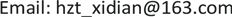Received: Jun. 4th, 2018; accepted: Jun. 20th, 2018; published: Jun. 27th, 2018ABSTRACT

This paper considers the consensus problem in discrete time multi-agent systems. By using variable step particle swarm algorithm, the coupling parameters among agents are locally self-tuned by least-mean square (LMS) algorithm, without using any global information. In this process, each agent minimizes a local cost function dependent on the error between the agent state and the average of neighbors’ states. Provided that the network graph is strongly connected, it is shown that for each agent, the sequence of coupling parameters is convergent, and all agent states converge toward the same constant value. Finally, the proposed algorithm is verified by simulation under different topologies.

Keywords:Multi-Agent Systems, Consensus, Self-Tuning, Least Mean Squares MethodCopyright © 2018 by authors and Hans Publishers Inc.

This work is licensed under the Creative Commons Attribution International License (CC BY).

http://creativecommons.org/licenses/by/4.0/1. 引言

2. 预备知识以及问题描述

2.1. 图论基础

2.2. 问题描述

${x}_{i}\left(t+1\right)={x}_{i}\left(t\right)+{\beta }_{i}{u}_{i}\left(t\right),\text{\hspace{0.17em}}\text{\hspace{0.17em}}i=1,\cdots ,N$ (1)

${x}_{i}\left(t\right)\in R$${u}_{i}\left(t\right)\in R$ 分别表示智能体在离散时间 $t=0,1,2,\cdots$ 时的状态和控制输入。 ${\beta }_{i}$ 表示第i个智能体的输入增益。假设 ${\beta }_{i}$ 的符号已知，但是它的大小是一个未知数。公式(1)可以被视为一组连续时间多智能体系统的离散版本  。

${u}_{i}\left(t\right)=\sum _{j\in {N}_{i}}{\theta }_{ij}\left(t\right)\left({x}_{j}\left(t\right)-{x}_{i}\left(t\right)\right)$ (2)

${\theta }_{ij}\left(t\right)$ 是控制参数，也是要进行估计的数值，以便智能体的状态 ${x}_{i}\left(t\right)$ 收敛到相同的值。例如，

$\underset{t\to \infty }{\mathrm{lim}}{x}_{i}\left(t\right)={x}_{c},\forall i\in V,{x}_{c}\in R$ (3)

${x}_{c}$ 为多智能体系统的状态变量达到一致的数值。在本文中，提出了基于LMS的变步长算法自适应地调整参数 ${\theta }_{ij}\left(t\right)$ 。定义参数向量：

${\theta }_{i}{\left(t\right)}^{\text{T}}=\left[{\theta }_{i1}\left(t\right){l}_{i1},\cdots ,{\theta }_{iN}\left(t\right){l}_{iN}\right],\forall i\in v$ (4)

${l}_{ij}=\left\{\begin{array}{l}1\text{\hspace{0.17em}}\text{\hspace{0.17em}}\text{\hspace{0.17em}}\text{\hspace{0.17em}}j\in {N}_{i}\\ 0\text{\hspace{0.17em}}\text{\hspace{0.17em}}\text{\hspace{0.17em}}\text{\hspace{0.17em}}其他\end{array}$

${u}_{i}\left(t\right)={\theta }_{i}{\left(t\right)}^{\text{T}}{\phi }_{i}\left(t\right)$ (5)

${\phi }_{i}\left(t\right)$ 是信号向量，定义如下：

${\phi }_{i}{\left(t\right)}^{\text{T}}=\left[{\epsilon }_{i1}\left(t\right){l}_{i1},\cdots ,{\epsilon }_{iN}\left(t\right){l}_{iN}\right]$ (6)

${\epsilon }_{ij}\left(t\right)={x}_{j}\left(t\right)-{x}_{i}\left(t\right)$ (7)

${J}_{i}\left({\theta }_{i}\right)=\frac{1}{2}{\left({x}_{i}\left(t+1\right)-{\overline{x}}_{i}\left(t+1\right)\right)}^{2}$ (8)

${\overline{x}}_{i}\left(t+1\right)=\frac{1}{1+N}\sum _{j\in {N}_{i}}{x}_{j}\left(t\right),{\overline{N}}_{i}={N}_{i}\cup \left\{i\right\}$ (9)

${\theta }_{i}\left(t+1\right)={\theta }_{i}\left(t\right)-{\mu }_{i}\mathrm{sgn}\left({\beta }_{i}\right)\frac{{\phi }_{i}\left(t\right){e}_{i}\left(t+1\right)}{{r}_{i}\left(t\right)}$ (10)

${r}_{i}\left(t\right)=1+{‖{\phi }_{i}\left(t\right)‖}^{2}$ (11)

${e}_{i}\left(t+1\right)={x}_{i}\left(t+1\right)-{\overline{x}}_{i}\left(t+1\right)$ (12)

${x}_{i}\left(t+1\right)={x}_{i}\left(t\right)+{\beta }_{i}{\theta }_{i}{\left(t\right)}^{\text{T}}{\phi }_{i}\left(t\right)$ (13)

$\frac{\partial {J}_{i}\left({\theta }_{i}\right)}{\partial {\theta }_{i}\left(t\right)}={e}_{i}\left(t+1\right)\frac{\partial {x}_{i}\left(t+1\right)}{\partial {\theta }_{i}\left(t\right)}={\beta }_{i}{\phi }_{i}\left(t\right){e}_{i}\left(t+1\right)$ (14)

$x\left(t+1\right)=W\left(t\right)x\left(t\right)$ (15)

$x{\left(t\right)}^{\text{T}}=\left[{x}_{1}\left(t\right),{x}_{2}\left(t\right),\cdots ,{x}_{N}\left(t\right)\right]$ (16)

$W\left(t\right)\in {R}^{N×N}$ 是耦合权重矩阵，定义如下:

$W\left(t\right)=\left[{w}_{ij}\right],{w}_{ij}\left(t\right)=\left\{\begin{array}{l}{\beta }_{i}{\theta }_{ij}\left(t\right),\text{\hspace{0.17em}}\text{\hspace{0.17em}}\text{\hspace{0.17em}}\text{\hspace{0.17em}}\text{\hspace{0.17em}}\text{\hspace{0.17em}}\text{\hspace{0.17em}}\text{\hspace{0.17em}}\text{\hspace{0.17em}}\text{\hspace{0.17em}}\text{\hspace{0.17em}}\text{\hspace{0.17em}}j\in {N}_{i}\\ 1-\sum _{K\in {N}_{i}}{\beta }_{i}{\theta }_{ij}\left(t\right),\text{\hspace{0.17em}}\text{\hspace{0.17em}}\text{\hspace{0.17em}}j=i\\ 0,\text{\hspace{0.17em}}\text{\hspace{0.17em}}\text{\hspace{0.17em}}\text{\hspace{0.17em}}\text{\hspace{0.17em}}\text{\hspace{0.17em}}\text{\hspace{0.17em}}\text{\hspace{0.17em}}\text{\hspace{0.17em}}\text{\hspace{0.17em}}\text{\hspace{0.17em}}\text{\hspace{0.17em}}\text{\hspace{0.17em}}\text{\hspace{0.17em}}\text{\hspace{0.17em}}\text{\hspace{0.17em}}\text{\hspace{0.17em}}\text{\hspace{0.17em}}\text{\hspace{0.17em}}\text{\hspace{0.17em}}\text{\hspace{0.17em}}\text{\hspace{0.17em}}其他\end{array}$ (17)

2.3. 相关证明

${\overline{x}}_{i}\left(t+1\right)={a}_{i}^{\text{T}}x\left(t\right)$ (18)

${a}_{i}^{\text{T}}=\left[{a}_{i1},\cdots ,{a}_{iN}\right],{a}_{ij}=\left\{\begin{array}{l}\frac{1}{1+{N}_{i}}\text{\hspace{0.17em}}\text{\hspace{0.17em}}j\in {N}_{i},j=i\\ 0\text{\hspace{0.17em}}\text{\hspace{0.17em}}\text{\hspace{0.17em}}\text{\hspace{0.17em}}\text{\hspace{0.17em}}\text{\hspace{0.17em}}\text{\hspace{0.17em}}\text{\hspace{0.17em}}\text{\hspace{0.17em}}\text{\hspace{0.17em}}\text{\hspace{0.17em}}\text{\hspace{0.17em}}\text{\hspace{0.17em}}\text{\hspace{0.17em}}其他\end{array}$ (19)

$e{\left(t\right)}^{\text{T}}=\left[{e}_{i}\left(t\right),\cdots ,{e}_{N}\left(t\right)\right]$ (20)

$x\left(t+1\right)=Ax\left(t\right)+e\left(t+1\right)$ (21)

$A={A}_{1}+l{y}_{A}^{\text{T}},\text{\hspace{0.17em}}\text{\hspace{0.17em}}\text{\hspace{0.17em}}l{t}_{A}^{\text{T}}=1$ (22)

$x\left(t+1\right)={A}_{1}x\left(t\right)+l{y}_{A}^{\text{T}}x\left(t\right)+e\left(t+1\right)$ (23)

$H\left({q}^{-1}\right)={\left(I-{q}^{-1}{A}_{1}\right)}^{-1}$ (24)

$x\left(t+1\right)=l{y}_{A}^{\text{T}}x\left(t\right)+H\left({q}^{-1}\right)e\left(t+1\right)$ (25)

${\varphi }_{i}\left(t\right)=x\left(t\right)-{x}_{i}\left(t\right)l,i\in v$ (26)

${\varphi }_{i}\left(t+1\right)=l{y}_{A}^{\text{T}}\left(x\left(t\right)-l{x}_{i}\left(t\right)\right)+l{y}_{A}^{\text{T}}l{x}_{i}\left(t\right)-l{x}_{i}\left(t+1\right)+H\left({q}^{-1}\right)e\left(t+1\right)$ (27)

${\varphi }_{i}\left(t+1\right)=l{y}_{A}^{\text{T}}{\varphi }_{i}\left(t\right)+l\left[{x}_{i}\left(t\right)-{x}_{i}\left(t+1\right)\right]+H\left({q}^{-1}\right)e\left(t+1\right)$ (28)

${\overline{x}}_{i}\left(t+1\right)={x}_{i}\left(t\right)+{b}_{i}^{\text{T}}{\varphi }_{i}\left(t\right)$ (29)

${b}_{i}^{\text{T}}=\left[{b}_{i1},\cdots ,{b}_{iN}\right],{b}_{ij}=\left\{\begin{array}{l}\frac{1}{1+{N}_{i}}\text{\hspace{0.17em}}\text{\hspace{0.17em}}j\in {N}_{i}\\ 0\text{\hspace{0.17em}}\text{\hspace{0.17em}}\text{\hspace{0.17em}}\text{\hspace{0.17em}}\text{\hspace{0.17em}}\text{\hspace{0.17em}}\text{\hspace{0.17em}}\text{\hspace{0.17em}}\text{\hspace{0.17em}}\text{\hspace{0.17em}}\text{\hspace{0.17em}}其他\end{array}$ (30)

${e}_{i}\left(t+1\right)={x}_{i}\left(t+1\right)-{x}_{i}\left(t\right)-{b}_{i}^{\text{T}}{\varphi }_{i}\left(t\right)$ (31)

${\varphi }_{i}\left(t+1\right)={Q}_{i}{\varphi }_{i}\left(t\right)-l{e}_{i}\left(t+1\right)+H\left({q}^{-1}\right)e\left(t+1\right)$ (32)

${Q}_{i}=l{\left({y}_{A}-{b}_{i}\right)}^{\text{T}}$ (33)

${b}_{i}^{\text{T}}=\left[{b}_{i1},\cdots ,{b}_{iN}\right],{b}_{ij}=\left\{\begin{array}{l}\frac{1}{1+{N}_{i}}\text{\hspace{0.17em}}\text{\hspace{0.17em}}j\in {N}_{i}\\ 0\text{\hspace{0.17em}}\text{\hspace{0.17em}}\text{\hspace{0.17em}}\text{\hspace{0.17em}}\text{\hspace{0.17em}}\text{\hspace{0.17em}}\text{\hspace{0.17em}}\text{\hspace{0.17em}}\text{\hspace{0.17em}}\text{\hspace{0.17em}}其他\end{array}$ (34)

${\rho }_{1}={l}^{\text{T}}\left({y}_{A}-{b}_{i}\right)=1-{l}^{\text{T}}{b}_{i}=1-{\sum }_{k=1}^{N}{b}_{ik}=1-\frac{{N}_{i}}{1+{N}_{i}}<1$ 因此存在正常数 ${c}_{0}$ ，使得对于所有的 $k\ge 0$$‖{Q}_{i}^{k}‖\le {c}_{0}{\rho }_{1}^{k},0<{\rho }_{1}<1$

${\varphi }_{i}\left(t+1\right)={Q}_{i}^{t+1}{\varphi }_{i}\left(0\right)+\sum _{k=0}^{t}{Q}_{i}^{t-k}\left[H\left({q}^{-1}\right)e\left(k+1\right)-l{e}_{i}\left(k+1\right)\right]$ (35)

${‖{\varphi }_{i}\left(t+1\right)‖}^{2}\le {c}_{1}{\rho }_{1}^{k}+{c}_{2}\sum _{k=0}^{t}{\rho }_{1}^{t-k}{‖e\left(k+1\right)‖}^{2}$ (36)

$\sum _{t=0}^{n}{‖{\varphi }_{i}\left(t+1\right)‖}^{2}\le {c}_{1}+{c}_{2}\sum _{t=0}^{n}{‖e\left(t+1\right)‖}^{2},\forall n\ge 0$ (37)

${e}_{i}\left(t+1\right)={\stackrel{˜}{\theta }}_{i}\left(t\right){\phi }_{i}\left(t\right)$ (38)

${e}_{i}\left(t+1\right)$ 由公式(12)定义，并且：

${\stackrel{˜}{\theta }}_{i}\left(t\right)={\beta }_{i}{\theta }_{i}\left(t\right)-{a}_{i}$ (39)

${\stackrel{˜}{\theta }}_{i}\left(t+1\right)={\stackrel{˜}{\theta }}_{i}\left(t\right)-\frac{{\mu }_{i}|{\beta }_{i}|}{{r}_{i}\left(t\right)}{\phi }_{i}\left(t\right){e}_{i}\left(t+1\right)$ (40)

${V}_{i}\left(t+1\right)={V}_{i}\left(t\right)-\frac{2{\mu }_{i}|{\beta }_{i}|}{{r}_{i}\left(t\right)}{\stackrel{˜}{\theta }}_{i}\left(t\right){\phi }_{i}\left(t\right)e{}_{i}\left(t+1\right)+\frac{{\left({\mu }_{i}{\beta }_{i}\right)}^{2}}{{r}_{i}\left(t\right)}\cdot \frac{{\phi }_{i}{\left(t\right)}^{2}}{{r}_{i}\left(t\right)}{e}_{i}{\left(t+1\right)}^{2}$ (41)

${V}_{i}\left(t+1\right)\le {V}_{i}\left(t\right)-2{\mu }_{i}|{\beta }_{i}|\left(1-\frac{{\mu }_{i}|{\beta }_{i}|}{2}\right)\sum _{t=0}^{n}\frac{{e}_{i}{\left(t+1\right)}^{2}}{{r}_{i}\left(t\right)}$ (42)

${V}_{i}\left(n+1\right)+2{\mu }_{i}|{\beta }_{i}|\left(1-\frac{{\mu }_{i}|{\beta }_{i}|}{2}\right)\sum _{t=0}^{n}\frac{{e}_{i}{\left(t+1\right)}^{2}}{{r}_{i}\left(t\right)}\le {V}_{i}\left(0\right)$ (43)

$\sum _{t=0}^{n}\frac{{e}_{i}{\left(t+1\right)}^{2}}{{r}_{i}\left(t\right)}\le {c}_{3}<\infty ,i\in v$ (44)

$\overline{r}\left(t\right)=\sum _{i=1}^{N}\underset{1\le \tau \le t}{\mathrm{max}}{r}_{i}\left(\tau \right)$ (45)

$\sum _{t=0}^{n}\frac{{‖e\left(t+1\right)‖}^{2}}{\overline{r}\left(t\right)}\le {c}_{4}\le \infty$ (46)

$\sum _{t=0}^{n}{‖e\left(t+1\right)‖}^{2}\le {c}_{5}<\infty$ (47)

$\underset{n\to \infty }{\mathrm{lim}}\frac{1}{\overline{r}\left(n\right)}{‖e\left(t+1\right)‖}^{2}=0$ (48)

$\overline{r}\left(n\right)\le {c}_{6}+{c}_{7}\sum _{t=0}^{n}{‖e\left(t+1\right)‖}^{2}$ (49)

$\sum _{t=0}^{n}{‖e\left(t+1\right)‖}^{2}\le {c}_{8}<\infty$ (50)

$\sum _{t=0}^{n}{‖{\phi }_{i}\left(t\right)‖}^{2}\le \sum _{t=0}^{n}{‖{\varphi }_{i}\left(t\right)‖}^{2}\le {c}_{9}\le \infty$ (51)

$\underset{t\to \infty }{\mathrm{lim}}\left(x\left(t\right)-{x}_{i}\left(t\right)l\right)=0,i\in v$ (52)

3. 仿真与分析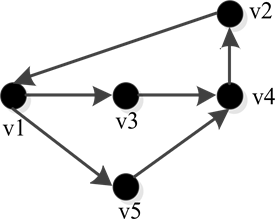Figure 1. A topology with a span ning treeTable 1. The initial positions and velocities of the agentsFigure 2. The amplitude of velocities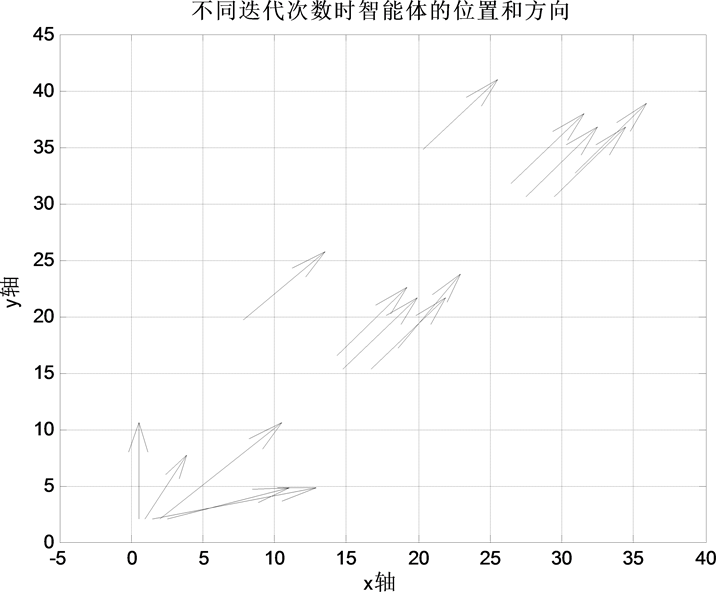Figure 3. Positions and directions of agents at different iterations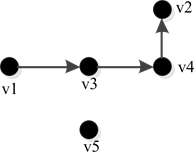Figure 4. A topology without a span ning tree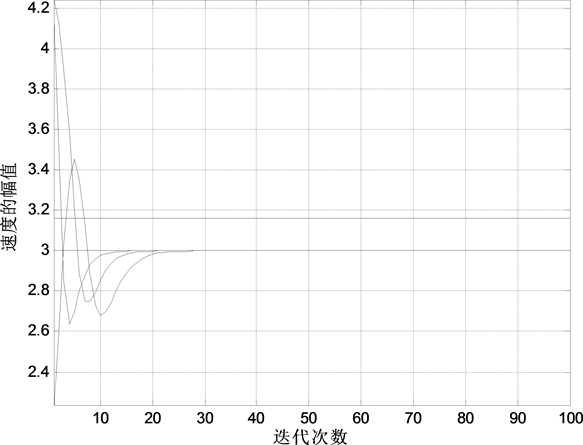Figure 5. The amplitude of velocities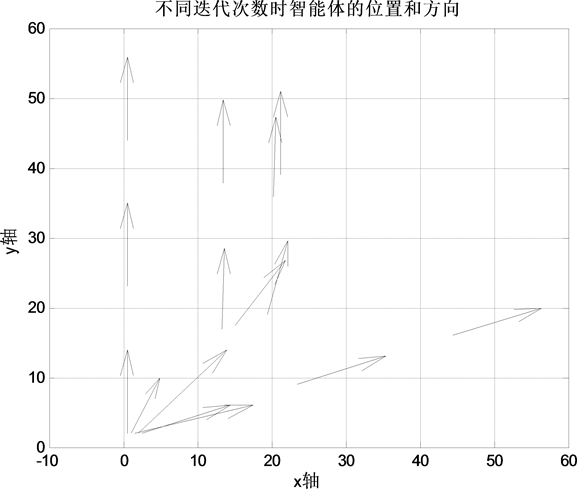Figure 6. Positions and directions of agents at different iterations

4. 结论

Variable Step Self-Tuning Consensus of Multi-Agent Systems with Least Mean Squares Method[J]. 计算机科学与应用, 2018, 08(06): 877-887. https://doi.org/10.12677/CSA.2018.86097

1. 1. Olfati-Saber, R. and Murray, R.M. (2004) Consensus Problems in Networks of Agents with Switching Topology and Time-Delays. IEEE Transactions on Automatic Control, 49, 1520-1533. https://doi.org/10.1109/TAC.2004.834113

2. 2. Ren, W. and Beard, R.W. (2005) Consensus Seeking in Mul-ti-Agent Systems under Dynamically Changing Interaction Topologies. IEEE Transactions on Automatic Control, 50, 655-661. https://doi.org/10.1109/TAC.2005.846556

3. 3. 李静, 方正. 分数阶非线性多智能体系统的一致性研究[J]. 计算机工程与应用, 2016, 52(23): 63-67.

4. 4. Li, T. and Zhang. J.F. (2010) Consensus Conditions of Mul-ti-Agent Systems with Time-Varying Topologies and Stochastic Communication Noises. IEEE Transactions on Auto-matic Control, 55, 2043-2057. https://doi.org/10.1109/TAC.2010.2042982

5. 5. Dong, X.W., Xi, J.X., Shi, Z.Y., et al. (2013) Practical Consen-sus for High-Order Linear Time-Invariant Swarm Systems with Interaction Uncertainties, Time-Varying Delays and Ex-ternal Disturbances. International Journal of Systems Science, 44, 1843-1856. https://doi.org/10.1080/00207721.2012.670296

6. 6. Jadbabaie, A., Lin, J. and Morse, A.S. (2003) Coordination of Groups of Mobile Autonomous Agents Using Nearest Neighbor Rules. IEEE Transactions on Automatic Control, 48, 988-1001. https://doi.org/10.1109/TAC.2003.812781

7. 7. 王品, 姚佩阳. 具有不同输入时延的二阶多智能体系统一致性[J]. 复杂系统与复杂性科学, 2016, 13(4): 102-107.

8. 8. Bai, H., Arcak, M. and Wen, J. (2011) Coopera-tive Control Design: A Systematic-Passivity Based Approach. Springer-Verlag, New York. https://doi.org/10.1007/978-1-4614-0014-1

9. 9. Ma, T.D., Zhang, L.Y. and Gu, Z.Y. (2016) Further Studies on Impulsive Consensus of Multi-Agent Nonlinear Systems with Control Gain Error. Neurocomputing, 190, 140-146. https://doi.org/10.1016/j.neucom.2016.01.024

10. 10. 袁玩贵, 屈百达. 受干预多智能体系统的一致性分析及控制[J]. 控制与决策, 2016, 31(1): 187-192.

11. 11. Cao, Y., Yu, W., Ren, W. and Chen, G. (2013) An Overview of Recent Progress in the Study of Distributed Multi-Agent Coordination. IEEE Transactions on Industrial Informatics, 9, 427-438. https://doi.org/10.1109/TII.2012.2219061

12. 12. Zhou, J., Lu, J. and Lü, J. (2006) Adaptive Synchronization of an Uncertain Complex Dynamical Network. IEEE Transactions on Automatic Control, 51, 652-656. https://doi.org/10.1109/TAC.2006.872760

13. 13. Zhou, J., Lu, J. and Lü, J. (2008) Pinning Adaptive Synchroniza-tion of a General Complex Dynamical Network. Automatica, 44, 996-1003. https://doi.org/10.1016/j.automatica.2007.08.016

14. 14. DeLellis, P., diBernardo, M. and Garofalo, F. (2009) Novel Decentralized Adaptive Strategies for Synchronization of Complex Networks. Automatica, 45, 1312-1318. https://doi.org/10.1016/j.automatica.2009.01.001

15. 15. Das, A. and Lewis, F. (2010) Distributed Adaptive Control for Synchronization of Unknown Nonlinear Networked Systems. Automatica, 46, 2014-2021. https://doi.org/10.1016/j.automatica.2010.08.008

16. 16. Yu, H. and Xia, X. (2012) Adaptive Consensus for Mul-ti-Agents in Networks with Jointly Connected Topologies. Automatica, 48, 1783-1790. https://doi.org/10.1016/j.automatica.2012.05.068

17. 17. Li, Z., Ren, W., Liu, X. and Xie, L. (2013) Distributed Con-sensus of Linear Multi-Agent Systems with Adaptive Dynamic Protocols. Automatica, 49, 1986-1995. https://doi.org/10.1016/j.automatica.2013.03.015

18. 18. Radenkovic, M. and Bose, T. (2015) On Multi-Agent Self-Tuning Consensus. Pergamon Press, Inc., Oxford.

19. 19. 张清华. 图论及其应用[M]. 北京: 清华大学出版社, 2013: 2-4.

20. 20. 吴永红, 刘敬贤. 多智能体网络一致性协同控制理论及应用[M]. 北京: 科学出版社, 2013.

21. 21. 李霄剑, 王永, 陈绍青, 等. 一种方向优化最小均方算法[J]. 电子与信息学报, 2014, 36(6): 1348-1354.